# Laplace equation, numerical methods

(diff) ← Older revision | Latest revision (diff) | Newer revision → (diff)

Methods that replace the original boundary value problem by a discrete problem containing a finite numberof unknows, such that if one finds a solution of the latter with suitable accuracy, this enables one to determine the solution of the original problem with given accuracy;depends onand tends to infinity as.

In the case ofspatial variables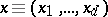, the Laplace equation has the form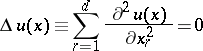and is the homogeneous Poisson equation. Boundary value problems for the Laplace equation are special cases of boundary value problems for the Poisson equation and more general equations of elliptic type (see ), and numerical methods for solving boundary value problems for equations of elliptic type (see , ) comprise many numerical methods for the Laplace equation. The specific character of the Laplace equation makes it possible to construct and use methods that have essentially better characteristics than methods for more general equations, although in practice one often prefers the simplicity of using general methods on a computer, rather than these possibilities.

The main numerical methods for equations of elliptic type are: projection-grid methods (finite-element methods) and difference methods. Both classes of methods are connected with the approximation of the original domainby a grid domain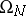containingnodes of the grid and the construction of a system of algebraic equations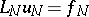(*)

for the values of the function defined at these nodes. In projection-grid methods, which are special cases of variational and projection methods, one uses the idea of approximation of the space of functions under consideration that contains the solution of the original problem by certain special finite-dimensional subspaces with given basis functions, and in the system (*) the vectorconsists of the coefficients of the expansion of the resulting approximation of the required solution with respect to the chosen basis. On the assumption that the solution of the original problem in a bounded domainof the plane has the form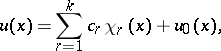where,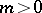, where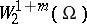denotes a Sobolev space, and the functions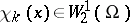are specified and reflect the asymptotic behaviour of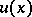close to singular points (corner points of the boundary and points where the type of boundary condition changes), for many types of domainsand mixed boundary value problems these methods make it possible, for example, to find the solutionto withinin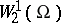in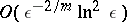arithmetic operations (see ), and in a number of more special cases the estimates of the computational work are lowered to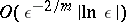and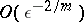.

In difference methods one commonly uses in one form or another an approximation of the derivatives by differences, and in the system (*) the vector consists of components that approximate the values of the solution at the nodes of the grid. The characteristics of the methods mentioned have been extensively studied for boundary value problems in bounded domainsof the plane. For example, for the Dirichlet condition, whereis the boundary ofand the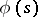are sufficiently smooth, on the basis of an improvement of the differentiability properties of the solution of the Laplace equation as one moves away fromone can construct the system (*) in such a way that the numberhas equal order as the number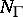of points onused to specifyto within, and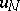can be found to withinin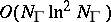arithmetic operations, and this makes it possible to find a solution of the original problem to withinat any fixed point of a strictly interior subdomain in a finite number of operations (see ). Methods of this type are asymptotically optimal; in the case when one uses, for example, simpler methods with a rectangular grid that have accuracy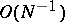, the number of operations required to findto withinis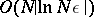;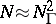(see ). Estimates of the error in the grid method for the Laplace equation have been studied in more detail (see , ); if there are singular points onit is advisable to use the special structure of the grid close to these points (see ). One often uses difference methods based on the approximation of certain integral characteristics for the Laplace equation (see ).

Fairly infrequently one uses collocation methods, in which the system (*) is obtained as a consequence of the original equation being satisfied at nodes of the grid and the assumption that an approximation to a solution of the original problem is sought in some finite-dimensional subspace. A special class of numerical methods for solving boundary value problems for the Laplace equation is based on the reduction of these problems to singular integral equations and the subsequent solution of the resulting integral equations by numerical methods (see , ).

How to Cite This Entry:
Laplace equation, numerical methods. Encyclopedia of Mathematics. URL: http://encyclopediaofmath.org/index.php?title=Laplace_equation,_numerical_methods&oldid=17252
This article was adapted from an original article by E.G. D'yakonov (originator), which appeared in Encyclopedia of Mathematics - ISBN 1402006098. See original article